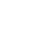# Understand the RxJS create Operator

Published 6 years ago
Updated 5 months ago

We have been using Observable.create() a lot in previous lessons, so let's take a closer look how does it work.

[00:00] We have been using observable.create a lot in previous lessons, so let's take a closer look at how does that work. Observable.create is actually the same as calling the constructor of the observable type. This here still behaves the same.

[00:14] The constructor takes just one argument, which is a function. That function takes one argument, that observer. Then you're supposed to call observer.next and error or complete, like we saw in previous lessons.

[00:26] If we would give a name for this function, what would that be? Here's a good suggestion -- subscribe. If we paste that function back here, give it a name, that's what's going to be passed here as the argument to the constructor. If you think there's some similarity between the function that we're calling and the function that we created, that's a correct guess.

[00:50] Here's an alternative way of writing this. Instead of giving three functions as arguments, we can give an object that has three fields -- next, error, and complete. Each of these are functions. This takes a x value, and then we will say console.log next x. The error is also a function. The complete is also a function.

[01:21] If we will give a name for this object actually, so we can extract this, give a name to it, let's say observer, it's an object. That's what we're going to pass down here. Now it's starting to make sense, how does Rx observables work. This function here is basically that function. We're calling that function given this object that has these three callbacks.

[01:50] If you want to see if it would work without all this RX machinery, this is really JavaScript. We don't have any more RX. We have a function subscribe that takes an object observer with some callbacks, and it's going to call those callbacks. As you can see, this all still works.

[02:08] Of course, it's useful to have the observable type because then it has all those nice operators that we saw and that we are also seeing new operators coming next. If you paid attention, then you're going to remember that in the subscribe, we had previously three functions here as argument. Instead of an object, as we have now, we had just these three functions.

[02:34] Also, the observable type, it converts these three functions into an observer object. Before it calls this, it will actually take these three functions and put labels in front of them like that, to create the observer object. It's normalizing it.

[02:54] That's in a nutshell what observable.create was. It's the same thing as the constructor. This is how the constructor works in a nutshell.~ 5 months ago

There are some typos in the transcriptLucas Minter
~ 5 months ago

There are some typos in the transcript

You are correct! I got those spelling mistakes and the images fixed. Thank you.